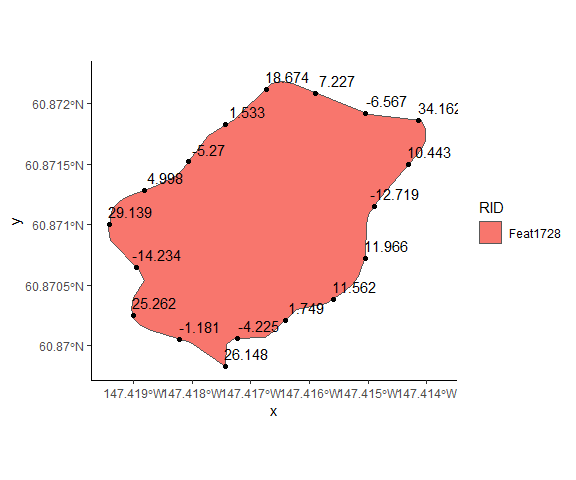# Introduction to Linear Feature Tools (rLFT)

## rLFT Introduction and Guide

This vignette will demonstrate how to use all of the linear feature tools available. rLFT makes use of linear referencing techniques and relies on the sf package to manipulate and extract data from given linear features.

## Example: Boundary Convexity Tool (BCT)

The BCT calculates the Raw Convexity value, Convexity Index, and Sinuosity at all user defined window-sizes simultaneously. The tool calculates one feature at a time and goes through the following process on each feature:

1. Adds m-values to the feature by using euclidean distance between x and y coordinates along the line. If you simply want to add measure values to your feature you can use the addMValues function.

2. Check if the feature is a polygon so we know to start calculating at the first vertex, or move to the first vertex that is equal to $$window / 2$$ from the beginning and the same distance from the ending vertex of the line.

3. While checking against the max m-value get three m-based points. Using these three points we create an arc and use that to calculate the convexity and sinuosity. For example we get the center point with the following equation: $$M + window/ 2$$ where M is the current measure value and window is the selected window size. The first point is just the measure value and the last point is $$M + window$$.

4. Format and store the data in a matirx.

5. Increment step-size by user-defined value and repeat steps 3-5 until max-m value is reached.

6. Return a data.frame with all the measurements (raw convexity, convexity index, sinuosity) for each feature and step size.

Below is an example:

library(rLFT)
#> Loading required package: Rcpp
#> Loading required package: sf
#> Linking to GEOS 3.6.1, GDAL 2.2.3, PROJ 4.9.3
library(ggplot2)
# read in example data
data("shpObject")
# to read in your own shp file use the sf package function st_read()
# choose any positive window size and step size. Here I use 50 for step size and 100 for window size.
outputTable <- bct(shpObject, step = 50, window = 100, ridName = "RID")
#>    user  system elapsed
#>    0.05    0.00    0.05
#>  "Features skipped due to size: "
#>    3  4  5  6  7  9 10 12 14 16 17 18 19 21 22 23 25 26 27 28 29 30 31 32 33
#>  34 35 36 37
outputTable$RID<- as.character(outputTable$RID)
# You can then look over the data using:
head(outputTable)
#>   FID      RID MidMeas WindowSize RawConvexity ConvexityIndex Sinuosity
#> 1   1 Feat1310       0        100       28.549          0.571     0.635
#> 2   1 Feat1310      50        100      -18.641         -0.373     0.578
#> 3   1 Feat1310     100        100      -19.891         -0.398     0.625
#> 4   1 Feat1310     150        100       24.944          0.499     0.623
#> 5   1 Feat1310     200        100        2.458          0.049     0.501
#> 6   1 Feat1310     250        100        9.879          0.198     0.512
#>   Midpoint_X Midpoint_Y
#> 1   354048.5    1226757
#> 2   354045.2    1226806
#> 3   354010.6    1226834
#> 4   353967.2    1226824
#> 5   353935.4    1226862
#> 6   353907.5    1226904

We can visualize the results of the function:

# Make the table spatially aware
outSF<- st_as_sf(outputTable, coords = c("Midpoint_X", "Midpoint_Y"), crs = st_crs(shpObject), stringsAsFactors = FALSE)

# plot a single island
ggplot(data = outSF[which(outSF$RID == as.character(shpObject$RID)), ]) +
geom_sf(data = st_cast(shpObject[15,], "POLYGON"), aes(fill = RID)) +
geom_sf() +
geom_sf_text(aes(label = RawConvexity), nudge_x = 20, nudge_y = 12) +
theme_classic()For a complete definition of the method, see: Albeke, S.E. et al. “Measuring boundary convexity at multiple spatial scales using a linear “moving window” analysis: an application to coastal river otter habitat selection.” Landscape Ecology 25 (2010): 1575-1587. linked phrase

### Example of ‘addMvalues’ function

You can use this function if you just want M values added to your sf object. Your sf object must be of type LINESTRING, MULTILINESTRING, POLYGON, or MULTIPOLYGON. The M Values are calculated by using the euclidean distance between the sf objects x and y coordinates (The sf library does not know how to handle M values so it treats them as Z values.)

data("shpObject")
monly <- shpObject

#Display coordinates
print("No M Values")
#>  "No M Values"
head(st_coordinates(monly))
#>             X       Y L1
#> [1,] 354048.5 1226757  1
#> [2,] 354047.0 1226759  1
#> [3,] 354047.0 1226760  1
#> [4,] 354046.9 1226798  1
#> [5,] 354044.8 1226808  1
#> [6,] 354041.0 1226816  1
# Assign M values to each vertex
monly <- addMValues(monly)

print("M Values Added")
#>  "M Values Added"
head(st_coordinates(monly))
#>             X       Y         M L1
#> [1,] 354048.5 1226757  0.000000  1
#> [2,] 354047.0 1226759  2.449931  1
#> [3,] 354047.0 1226760  3.720473  1
#> [4,] 354046.9 1226798 41.838002  1
#> [5,] 354044.8 1226808 51.931361  1
#> [6,] 354041.0 1226816 60.504098  1

## Planned Future Functionality

• Be able to take a set of points and snap them to the route layer and give the points the measure value of where they snap (snap = closest location on the route).